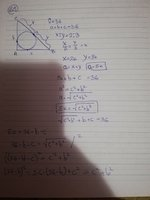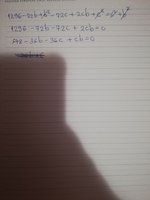# NEED HELP trinagle

#### Ana.stasia

##### New member
The question is:
Calculate all sides of a triangle which has a 90 degree angle and it's area if you know that the circle drawn inside the triangle touches the side opposite to the 90 degree angle so that it divides the side into two part whose ratio is 2:3 and if you know that thr sum of all sides is 36.

I tried to get a formula that would get me a ratio of sorts for the sides so I can just divide 36 by the ration. However I was unable to do that.
Here is everything I could come up with.

What am I not seeing?#### skeeter

##### Elite Member
the circle center, the incenter, occurs at the intersection of the angle bisectors of the triangle’s three angles

#### Ana.stasia

##### New member
the circle center, the incenter, occurs at the intersection of the angle bisectors of the triangle’s three angles
Okay, that would tell me that x=b-r and y=c-r which brings me to the conclusion that r+ 5k =18. What next?

#### Ana.stasia

##### New member
$$\displaystyle 10x + 2r = 36$$

$$\displaystyle (r+2x)^2 + (r+3x)^2 = (2x+3x)^2$$

try again ...

View attachment 22311
I got r+5k=18 which is the same thing as 2r+10x=36. It's only multiplied by two. I don't understand how (r+2x)2+(r+3x)2=(2x+3x)2
What is (2x+3x)2 ?

#### skeeter

##### Elite Member
Pythagoras

base leg = r + 3x

vertical leg = r + 2x

hypotenuse = 2x + 3x = 5x